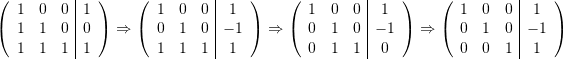### Direct Methods: Naive Gauss Elimination

### The Method

The naive Gauss elimination is a procedure in which the linear system of equations is manipulated such that the coefficients of the component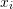are eliminated from equation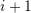to equation. It would then be possible to solve for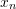using the last equation, and then backward substitute to find the remaining components. The procedure is divided into two steps, forward elimination followed by backward substitution. We will illustrate the process in the following example. Consider the system of equations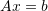defined as: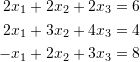Forward elimination: First, we will use the first equation to eliminate the coefficients of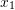in equation 2 and 3. By multiplying the first equation by -1 and adding it to the second equation we get: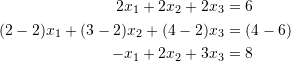which becomes: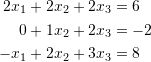By multiplying the first equation byand adding it to the third equation we get: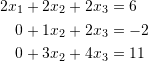We are now ready to eliminate the coefficient of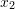from equation 3 by multiplying equation 2 by -3 and adding it to equation 3: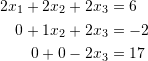Backward substitution: Solving the above system becomes very simple.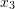is straightforward, it is equal to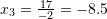.can be obtained from the second equation as: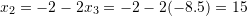and finally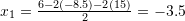. Therefore, the solution is: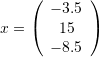Of course, as expected, if we multiply the original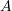bywe should get the matrix.

The Naive Gauss elimination method can be implemented in matrix form of dimensions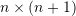as follows: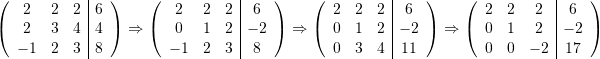The following code implements the above calculations in Mathematica

View Mathematica Code
A = {{2, 2, 2}, {2, 3, 4}, {-1, 2, 3}};
b = {6, 4, 8};
Aa = {{2, 2, 2, b[]}, {2, 3, 4, b[]}, {-1, 2, 3, b[]}};
Aa[] = Aa[] + -1*Aa[]
Aa[] = Aa[] + 1/2*Aa[]
Aa[] = Aa[] - 3 Aa[]
Aa // MatrixForm
x3 = Aa[[3, 4]]/Aa[[3, 3]]
x2 = (Aa[[2, 4]] - Aa[[2, 3]]*x3)/Aa[[2, 2]]
x1 = (Aa[[1, 4]] - Aa[[1, 2]]*x2 - Aa[[1, 3]]*x3)/Aa[[1, 1]]

View Python Code
import numpy as np
A = [[2, 2, 2], [2, 3, 4], [-1, 2, 3]]
b = [6, 4, 8]
Aa = np.array([[2, 2, 2, b], [2, 3, 4, b], [-1, 2, 3, b]])
Aa = Aa + -1*Aa
print("Aa:",Aa)
Aa = Aa + 1/2*Aa
print("Aa:",Aa)
Aa = Aa - 3*Aa
print("Aa:",Aa)
print("Aa:",Aa)
x3 = Aa[2, 3]/Aa[2, 2]
print("x3:",x3)
x2 = (Aa[1, 3] - Aa[1, 2]*x3)/Aa[1, 1]
print("x2:",x2)
x1 = (Aa[0, 3] - Aa[0, 1]*x2 - Aa[0, 2]*x3)/Aa[0, 0]
print("x1:",x1)


### Programming the Method

The following are the steps to program the Naive Gauss Elimination method. Assuming annumber of equations inunknowns of the form:

1. Form the combined matrix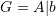2. Forward elimination: Use the pivot elements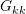on the row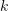as “Pivot” elements. Use the “Pivot” elements to eliminate the components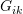withfrom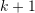to. This is done by iteratingfromtoand for each row, doing the operation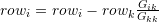.
3. Backward substitution: The elementwithrunning backwards fromto 1 can be found using the equation: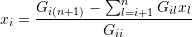The following is the code that relies on the “Sum” built-in function in Mathematica.

View Mathematica Code
(*Procedure*)
GaussElimination[A_, b_] :=
(n = Length[A];
G = Transpose[Insert[Transpose[A], b, n + 1]];
For[k = 1, k <= n, k++,
Do[G[[i]] = G[[i]] - G[[i, k]]/G[[k, k]]*G[[k]], {i, k + 1, n}]];
x = Table[0, {i, 1, n}];
For[i = n, i >= 1, i = i - 1,
x[[i]] = (G[[i, n + 1]] - Sum[G[[i, l]]*x[[l]], {l, i + 1, n}])/G[[i, i]]];
x)
(*An example*)
A = {{1, 2, 3, 5}, {2, 0, 1, 4}, {1, 2, 2, 5}, {4, 3, 2, 2}};
b = {-4, 8, 0, 10};
(*Applying the procedure to the example*)
N[GaussElimination[A, b]]
GaussElimination[A, b]

View Python Code
import numpy as np
# Procedure
def GaussElimination(A,b):
n = len(A)
G = (np.vstack([A.astype(np.float).T, b.astype(np.float)])).T
for k in range(n):
for i in range(k + 1, n):
G[i] = G[i] - G[i][k]/G[k][k]*G[k]
x = np.zeros(n)
for i in range(n-1,-1,-1):
x[i] = (G[i][n] - sum([G[i][l]*x[l] for l in range(i + 1, n)]))/G[i][i]
return x

# An example
A = np.array([[1, 2, 3, 5], [2, 0, 1, 4], [1, 2, 2, 5], [4, 3, 2, 2]])
b = np.array([-4, 8, 0, 10])
# Applying the procedure to the example
GaussElimination(A, b)


The following is the code that does not rely on the built-in function in Mathematica.

View Mathematica Code
(*Procedure*)
GaussElimination[A_, b_] := (n = Length[A];
G = Transpose[Insert[Transpose[A], b, n + 1]];
For[k = 1, k <= n, k++,
Do[G[[i]] = G[[i]] - G[[i, k]]/G[[k, k]]*G[[k]], {i, k + 1, n}]];
x = Table[0, {i, 1, n}];

For[i = n, i >= 1,
i = i - 1, (BB = 0; Do[BB = G[[i, l]]*x[[l]] + BB, {l, i + 1, n}];
x[[i]] = (G[[i, n + 1]] - BB)/G[[i, i]])];
x)
(*An example*)
A = {{1, 2, 3, 5}, {2, 0, 1, 4}, {1, 2, 2, 5}, {4, 3, 2, 2}};
b = {-4, 8, 0, 10};
(*Applying the procedure to the example*)
N[GaussElimination[A, b]]
GaussElimination[A, b]

View Python Code
import numpy as np
# Procedure
def GaussElimination(A,b):
n = len(A)
G = (np.vstack([A.astype(np.float).T, b.astype(np.float)])).T
for k in range(n):
for i in range(k + 1, n):
G[i] = G[i] - G[i][k]/G[k][k]*G[k]
x = np.zeros(n)
for i in range(n-1,-1,-1):
BB = 0
for l in range(i+1, n):
BB = G[i][l]*x[l] + BB
x[i] = (G[i][n] - BB)/G[i][i]
return x

# An example
A = np.array([[1, 2, 3, 5], [2, 0, 1, 4], [1, 2, 2, 5], [4, 3, 2, 2]])
b = np.array([-4, 8, 0, 10])
# Applying the procedure to the example
GaussElimination(A, b)


The following link provides the MATLAB code for implementing the Naive Gauss Elimination Method.

### Why Naive

The method described above is considered “naive” for two reasons. The first reason is that it fails to find a solution if a pivot element (one of the diagonal elements) is found to be zero. For example, consider the linear system: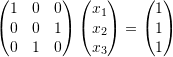This is really simple and the solution is just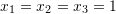. However, the code fails to find a solution because when the second row is used for elimination, the pivot element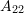is equal to zero, and so, an error is encountered. Try the above systems out and see the error that Mathematica spits out. This problem can be solved by simply exchanging rows 2 and 3. The same issue will be encountered for the following system: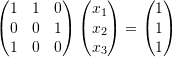In this case, one solution would be to rearrange the equations such that equation 3 would be equation 1. In general, the naive Gauss elimination code presented above can be augmented so that the pivot elements are chosen to be the largest elements in the column below. This augmentation is termed: “Partial Pivoting”. Partial Pivoting is performed by exchanging the rows. In this case, the order of the variablesstays the same. In rare cases, there could be a need to exchange the columns as well; a procedure termed “Complete Pivoting”, but this adds the complication of re-arranging the variables.

The second reason why it is called naive is that the method essentially produces an upper triangular matrix that can be easily solved. It is important to note that if the system is already a lower triangular matrix, then there is no need to employ the forward elimination procedure, because we can simply use forward substitution to find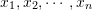in this order.
Consider the following system: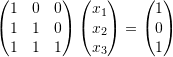It is straightforward that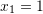,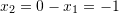, and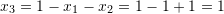. However, employing the naive Gauss elimination would conduct the following steps: